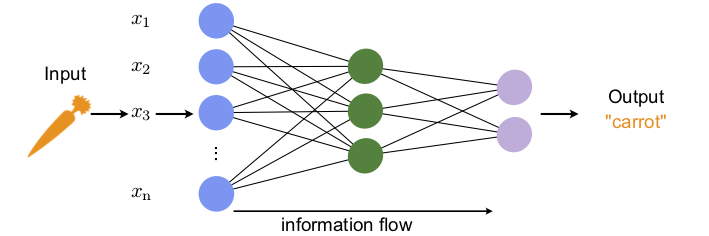# Supervised Learning with Neural Networks¶

In the previous chapter, we covered the basics of machine learning using conventional methods such as linear regression and principle component analysis. In the present chapter, we move towards a more complex class of machine learning models: neural networks. Neural networks have been central to the recent vast success of machine learning in many practical applications.

The idea for the design of a neural network model is an analogy to how biological organisms process information. Biological brains contain neurons, electrically activated nerve cells, connected by synapses that facilitate information transfer between neurons. The machine learning equivalent of this structure, the so-called artificial neural networks or neural networks in short, is a mathematical function developed with the same principles in mind. It is composed from elementary functions, the neurons, which are organized in layers that are connected to each other. To simplify the notation, a graphical representation of the neurons and network is used, see Fig. 12. The connections in the graphical representation means that the output from one set of neurons (forming one layer) serves as the input for the next set of neurons (the next layer). This defines a sense of direction in which information is handed over from layer to layer, and thus the architecture is referred to as a feed-forward neural network.

In general, an artificial neural network is simply an example of a variational non-linear function that maps some (potentially high-dimensional) input data to a desired output. Neural networks are remarkably powerful and it has been proven that under some mild structure assumptions they can approximate any smooth function arbitrarily well as the number of neurons tends to infinity. A drawback is that neural networks typically depend on a large amount of parameters. In the following, we will learn how to construct these neural networks and find optimal values for the variational parameters.

In this chapter, we are going to discuss one option for optimizing neural networks: the so-called supervised learning. A machine learning process is called supervised whenever we use training data comprising input-output pairs, in other words input with known correct answer (the label), to teach the network-required task.Fig. 12 Graphical representation of the basic neural network architecture.